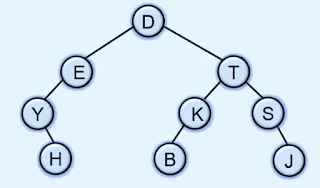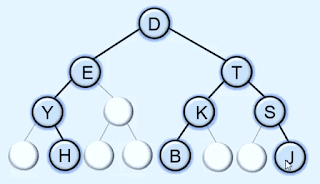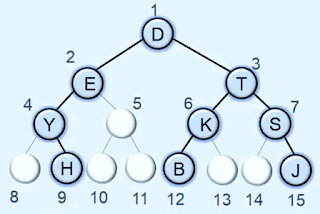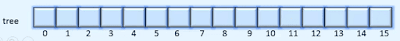# Array representation of Binary tree | Data structures

To represent a binary tree using array first we need to convert a binary tree into a full binary tree. and then we give the number to each node and store it into their respective locations.

let's take an example to understand how to represent a binary tree using an array.

to do this first we need to convert a binary tree into a full binary tree.here in the above example to convert this binary tree into a full binary tree we need to add nodes that don't have child nodes till the last level of the tree.So now the tree becomes a full binary tree. after that to represent it using an array we need to give the numbers to each and every node but level by level.after giving the number to each and every node now we need to create an array of size 15 + 1.after that store each one node in array in their respective index points. like D has number 1 then we store it in the array at index 1 and E has number 2 then we store it at index 2 in the array.so this is the array representation of a binary tree.

### Important terms to represent a binary tree into sequential order.

The root is always stored at index 1 in the array.

if any node is stored at K position then the left child of a node is stored at index 2k and the right child is stored at index 2K + 1 and the parent of a node is stored at floor(K/2) index.

Note: The size of an array to represent a binary tree of height H is equal to the maximum number of nodes possible in a binary tree of height H.

#### Program to implement binary tree using python programming

```from collections import deque

class Node:
def __init__(self, value):
self.info = value
self.lchild = None        self.rchild = None

class BinaryTree:
def __init__(self):
self.root = None
def is_empty(self):
return self.root is None
def display(self):
self._display(self.root, 0)
print()

def _display(self,p,level):
if p is None:
return        self._display(p.rchild, level+1)
print()

for i in range(level):
print(" ", end='')
print(p.info)
self._display(p.lchild, level+1)

def preorder(self):
self._preorder(self.root)
print()

def _preorder(self,p):
if p is None:
return        print(p.info, " ", end='')
self._preorder(p.lchild)
self._preorder(p.rchild)

def inorder(self):
self._inorder(self.root)
print()

def _inorder(self,p):
if p is None:
return        self._inorder(p.lchild)
print(p.info," ", end='')
self._inorder(p.rchild)

def postorder(self):
self._postorder(self.root)
print()

def _postorder(self,p):
if p is None:
return        self._postorder(p.lchild)
self._postorder(p.rchild)
print(p.info," ",end='')

def level_order(self):
if self.root is None:
print("Tree is empty")
return
qu = deque()
qu.append(self.root)

while len(qu) != 0:
p = qu.popleft()
print(p.info + " ", end='')
if p.lchild is not None:
qu.append(p.lchild)
if p.rchild is not None:
qu.append(p.rchild)

def height(self):
return self._height(self.root)

def _height(self,p):
if p is None:
return 0
hL = self._height(p.lchild)
hR = self._height(p.rchild)

if hL > hR:
return 1 + hL
else:
return 1 + hR

def create_tree(self):
self.root = Node('p')
self.root.lchild = Node('Q')
self.root.rchild = Node('R')
self.root.lchild.lchild = Node('A')
self.root.lchild.rchild = Node('B')
self.root.rchild.lchild = Node('X')

##########################
bt = BinaryTree()

bt.create_tree()

bt.display()
print()

print("Preorder : ")
bt.preorder()
print("")

print("Inorder : ")
bt.inorder()
print()

print("Postorder : ")
bt.postorder()
print()

print("Level order : ")
bt.level_order()
print()

print("Height of tree is ", bt.height())

```